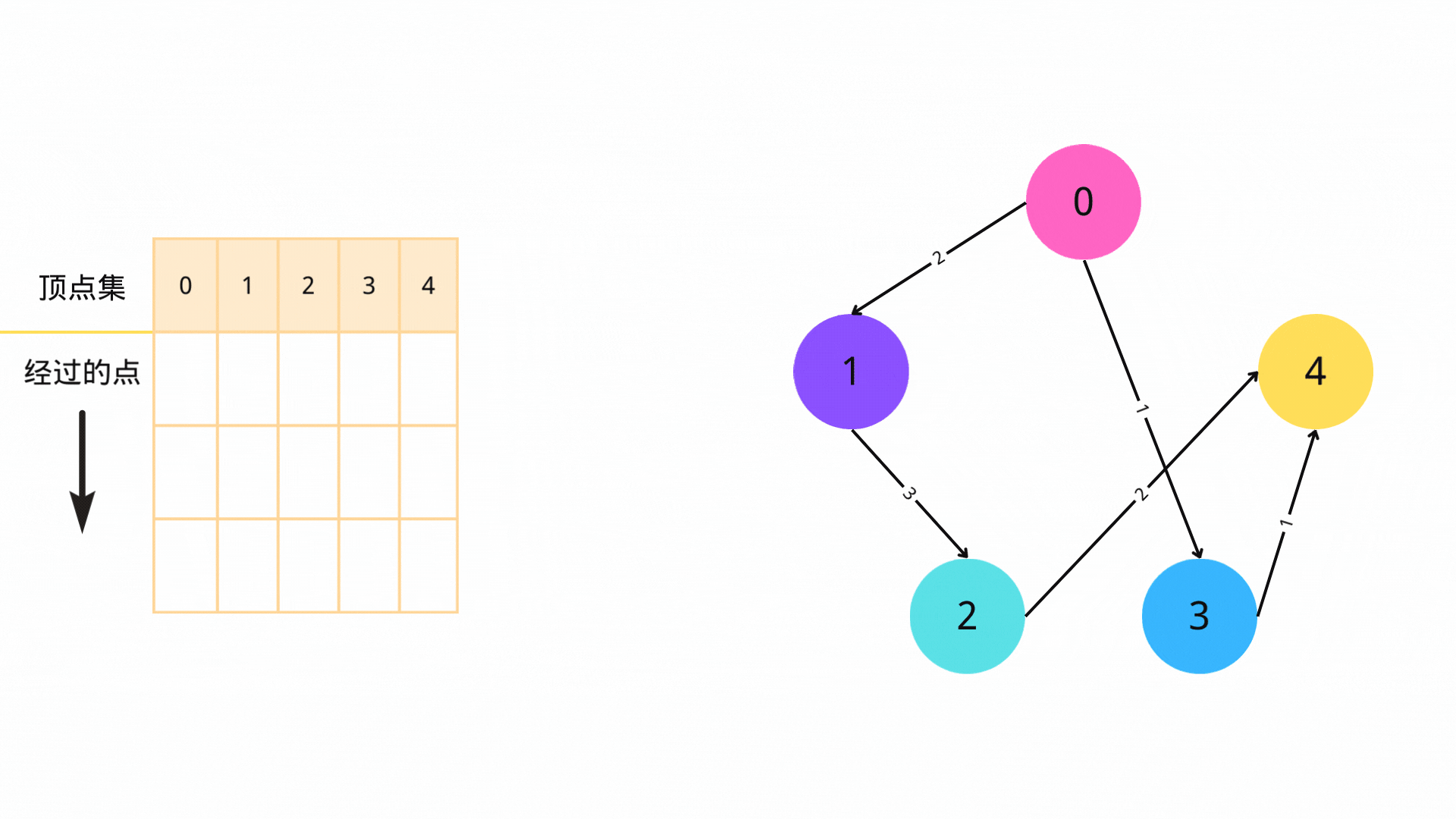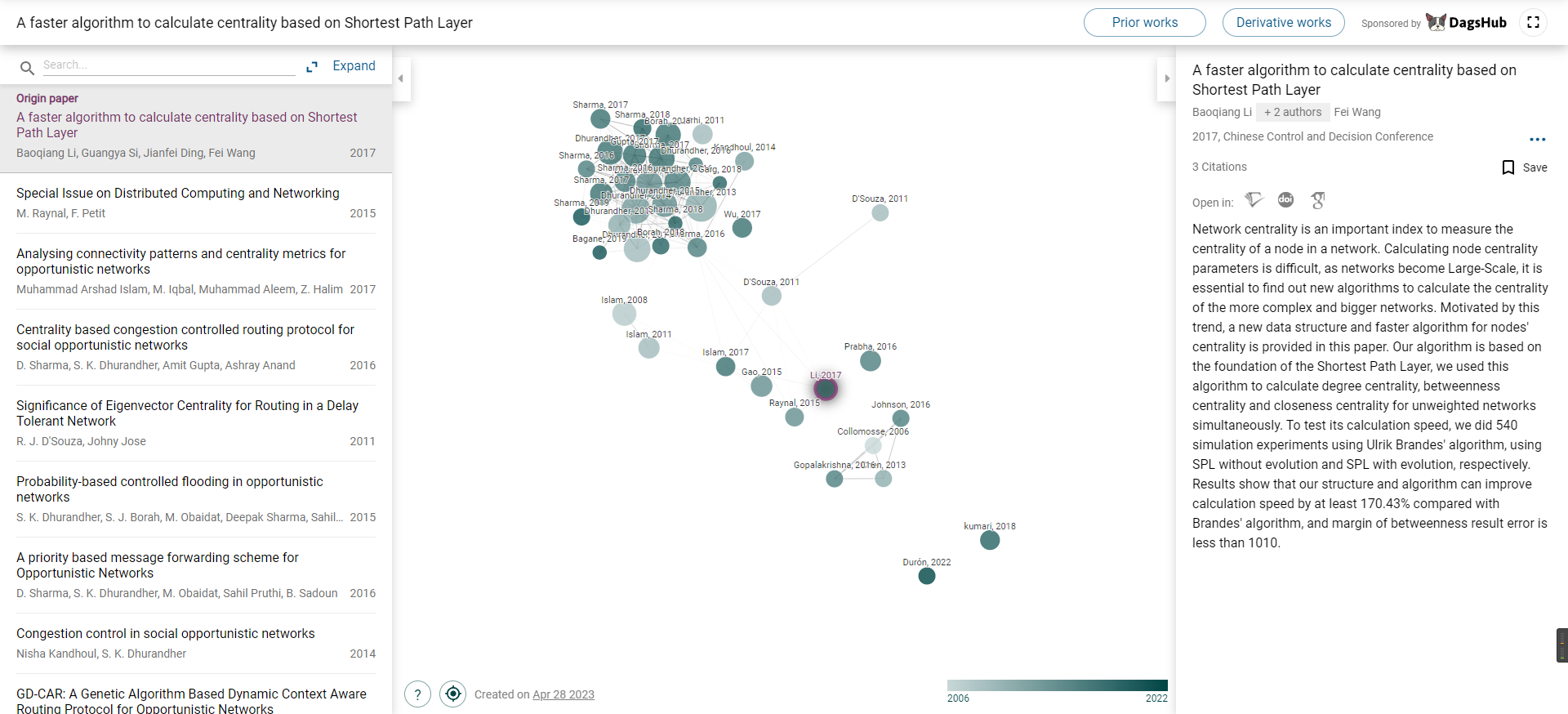# SPFA 算法：实现原理及其应用 原创

0收藏

@[toc]

## 一、前言

SPFA算法，全称为Shortest Path Faster Algorithm，是求解单源最短路径问题的一种常用算法，它可以处理有向图或者无向图，边权可以是正数、负数，但是不能有负环。

## 二、SPFA 算法

### 1、SPFA算法的基本流程

1. 初始化2. 迭代3. 循环

4. 判断

### 2、代码详解

``````import java.util.*;

class Graph {   // 图
private List<Vertex> vertices;  // 顶点集

public Graph() {
vertices = new ArrayList<Vertex>();
}

public void addVertex(Vertex v) {   // 添加顶点
}   // 添加顶点

public List<Vertex> getVertices() { // 返回顶点
return vertices;
}   // 获取顶点集
}

class Vertex {  // 点
private int id; // 点 id
private List<Edge> edges;   // 连接到该顶点的边
private int distance;   // 从源顶点到该顶点的最短距离，MAX_VALUE init
private boolean visited;    // 在图的遍历过程中是否访问过该顶点，false init

public Vertex(int id) {
this.id = id;
edges = new ArrayList<Edge>();
distance = Integer.MAX_VALUE;
visited = false;
}

public int getId() {    // 获取 id
return id;
}

public void addEdge(Edge e) {   // 将连接到该顶点边添加到列表中
}   // 添加图到边

public List<Edge> getEdges() {  // 获取连接到该顶点的边集
return edges;
}   // 获取图中边

public int getDistance() {  // 获取从源顶点到该顶点的最短距离
return distance;
}   // 获取源顶点到该顶点的最短距离

public void setDistance(int distance) { //设置最短距离
this.distance = distance;
}   // 设置源顶点到该顶点的最短距离

public boolean isVisited() {    // 获取在图的遍历过程中是否访问过该点
return visited;
}   // 获取图遍历过程中是否访问过该点

public void setVisited(boolean visited) {   // 设置在图的遍历过程中是否访问过该点
this.visited = visited;
}   // 设置图遍历过程中是否访问过该点
}

class Edge {    // 边
private Vertex source;  // 源顶点
private Vertex destination; // 目标顶点
private int weight; // 边的权重

public Edge(Vertex source, Vertex destination, int weight) {
this.source = source;
this.destination = destination;
this.weight = weight;
}

public Vertex getSource() { // 返回源顶点
return source;
}   // 获取源点

public Vertex getDestination() {    // 返回目标顶点
return destination;
}   // 获取目标顶点

public int getWeight() {    // 获取边的权重
return weight;
}   // 获取边的权重
}

// SPFA 算法
public class SPFA {
public static void spfa(Graph graph, Vertex source) {
// 初始化
Queue<Vertex> queue = new LinkedList<Vertex>(); // 初始化一个顶点队列，使用该队列来跟中需要处理的顶点
for (Vertex v : graph.getVertices()) {  // 初始化最短距离和是否访问过该点
v.setDistance(Integer.MAX_VALUE);
v.setVisited(false);
}

source.setDistance(0); // 将源顶点到自身的最短距离设为0

// 迭代
int count = 0;  // 用于检测图中的负环，count超过图中顶点的总数，抛出异常

// 查找从一个源顶点到图中所有其它顶点的最短路径
while (!queue.isEmpty()) {
Vertex u = queue.poll();    // 存储SPFA算法正在处理的顶点，poll 方法将下一个顶点从队列中取出
u.setVisited(false);    // 标记该顶点为未访问，以便在算法中再次对其处理

// 查找部分，循环遍历当前顶点 u 的所有边
for (Edge e : u.getEdges()) {
Vertex v = e.getDestination();  // 返回边 e 的目标顶点给 v
int distance = u.getDistance() + e.getWeight(); // 计算源顶点到目标顶点的距离
if (distance < v.getDistance()) {
v.setDistance(distance);    // 更新最短距离
if (!v.isVisited()) {   // 如果该顶点未被访问过
v.setVisited(true); // 标记该顶点已被访问
count++;    // 负环 + 1
if (count > graph.getVertices().size()) {   // 检查 SPFA 算法处理的顶点数是否大于图中顶点总数
throw new RuntimeException("Negative cycle detected");
}
}
}
}
}
}

public static void main(String[] args) {

// 构造图
Graph graph = new Graph();
// 构造顶点
Vertex s = new Vertex(0);
Vertex a = new Vertex(1);
Vertex b = new Vertex(2);
Vertex c = new Vertex(3);
Vertex d = new Vertex(4);
// 点加边
// 边加点

// 调用SPFA算法求解最短路径
spfa(graph, s);

// 输出结果
for (Vertex v :graph.getVertices()) {
System.out.println("Shortest distance from source to vertex " + v.getId() + " is " + v.getDistance());
}
}
}
``````

1. 初始化：将所有顶点的距离设置为正无穷，将源点的距离设置为0，将源点加入队列。
2. 迭代：从队列中取出一个顶点u，遍历它的所有邻居v。如果u到源点的距离加上u到v的边的权重小于v的距离，则更新v的距离，并将v加入队列中。如果v已经在队列中，则不需要再次添加。
3. 如果队列为空，则算法结束。如果队列非空，则回到步骤2。

SPFA算法的时间复杂度取决于负权边的数量。如果图中没有负权边，算法的时间复杂度是O(E)，其中E是边的数量。但是如果图中有负权边，算法的时间复杂度将达到O(VE)，其中V是顶点的数量，E是边的数量。因此，为了避免算法的时间复杂度变得非常高，应尽可能避免在图中使用负权边。

## 三、SPFA 算法已死 ？

• 可能会进入负环：SPFA 算法可以处理负权边，但是如果有负权环，算法将无法结束，因为每次都会沿着负权环一遍一遍地更新距离，导致算法陷入死循环。

• 时间复杂度不稳定：在最坏情况下，SPFA 算法的时间复杂度可以达到 \$O(VE)\$，其中 \$V\$ 和 \$E\$ 分别是图中的顶点数和边数。而在最好情况下，时间复杂度只有 \$O(E)\$。因此，SPFA 算法的时间复杂度是不稳定的。

• 存在更好的算法：对于单源最短路径问题，已经有更好的算法出现，如 Dijkstra 算法和 Bellman-Ford 算法。这些算法在时间复杂度和稳定性方面都比 SPFA 算法更优秀。